N 6.7 post test
First name *
Last name *
Homeroom *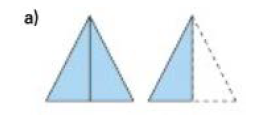For image a above, write an improper fraction and a mixed number. *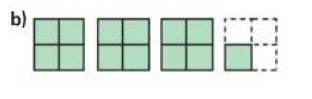For image b above, write an improper fraction and a mixed number. *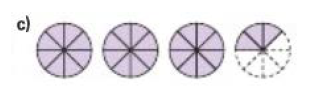For image c above, write an improper fraction and a mixed number. *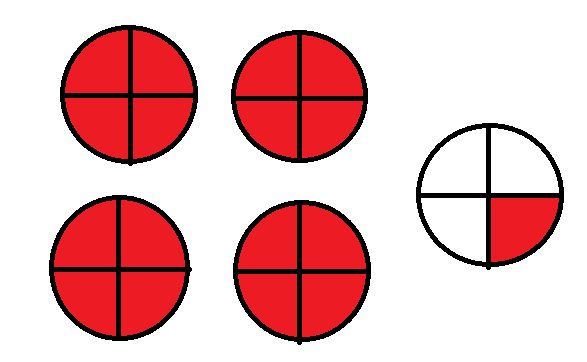Write an improper fraction and a mixed number for the image above, *
Write the following number as an improper fraction. 3 7/9 *
Write the following number as an improper fraction. 4 3/4 *
Write the following number as a mixed number. 8/5 *
Write the following number as a mixed number. 39/7 *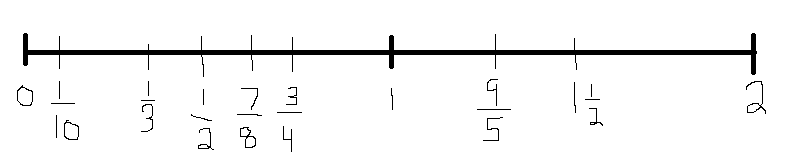Look at the above number line. 2 fractions are in the wrong places. Which ones are they? How do you know? *
Suppose you have 14 quarters. Do you have \$4? If not, how much money do you have? *